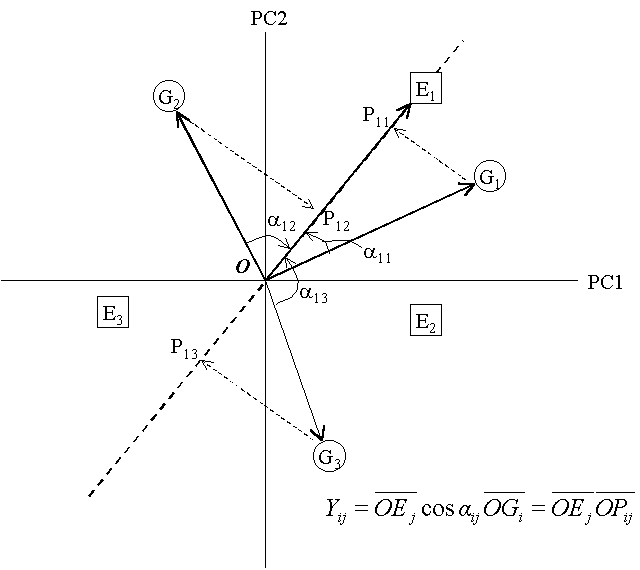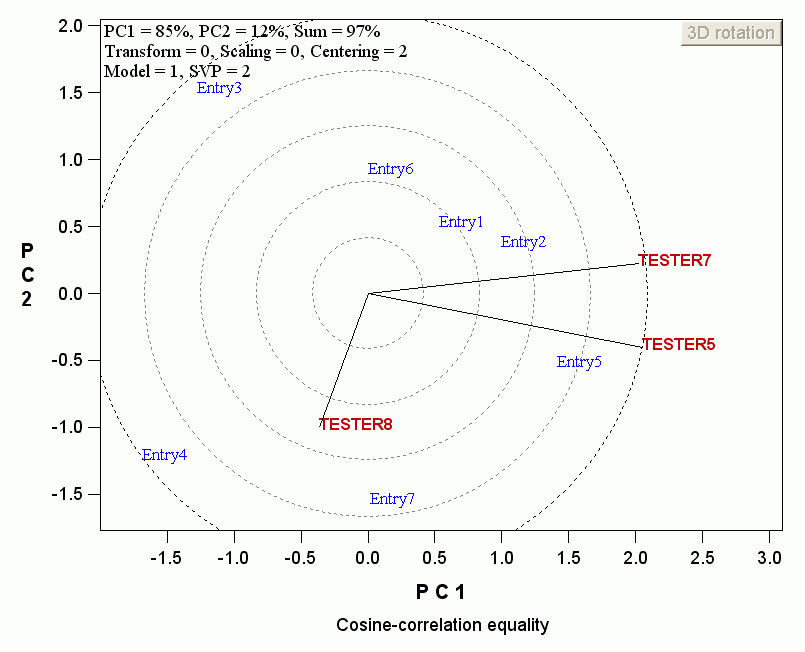Contents - Index

Principles of biplot analysis

The biplot has many utilities, and many utilities are based on the following two basic principles:

1. The inner-product propertyA biplot is a graphical displays of a two-way table. If the two-way table is 100% displayed by the biplot, then there is a strict trimetric relationship between the value of entry i in tester j, Yij,  and their coordinates in the biplot. Verbally, the value of Entry i in Tester j is the vector length of Entry i (OGi) multiplied by the vector length of Tester j (OEj) and multiplied by the cosine of the angle between Entry i and Tester j (aij). Many important interpretations of biplots are based on this principle.

2. The cosine-correlation equality

When the biplot is based on tester centered data (all GGE biplots are based on this type of centering), and when the tester-focused singular-value partitioning, i.e., SVP =2, is used, the correlation between two testers can be approximated by the cosine of the angle between them:

cos(aij) = rij

where aij is the angle between tester i and tester j, and rij is the correlation coefficient between tester i and tester j.

Examine the angles among the three testers in the following biplot and compare with the correlation coefficients among them in the table below. The correspondence should be near-perfect as the biplot explained 97% of the total variation.The correlation coefficients among the three testers are:

Correlation TESTER5 TESTER7 TESTER8

TESTER5   1   0.912 -0.177

TESTER7   0.912   1 -0.406

TESTER8 -0.177 -0.406 1

Important: The cosine-correlation equality principle is valid only when the above two conditions are met and when the biplot is drawn to scale.

For a more complete reference on the principles of biplot analysis, see, for example, Yan and Tinker (2006).